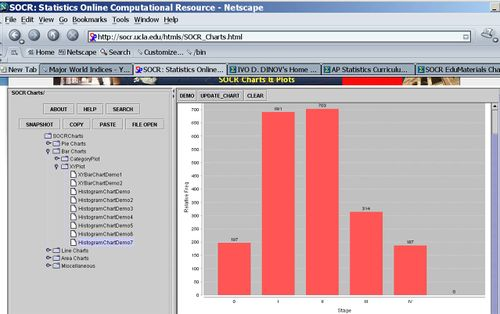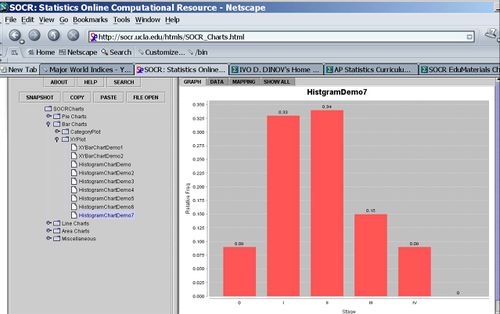# Difference between revisions of "AP Statistics Curriculum 2007 EDA Freq"

## General Advance-Placement (AP) Statistics Curriculum - Summarizing data with Frequency Tables

### Summarizing data with Frequency Tables & Histograms

There are two ways to describe a data set (sample from a population) - Pictorial Graphs or Tables of Numbers. Both are important for analyzing data.

### Definitions

• A frequency distribution is a display of the number (frequency) of occurrences of each value in a data set.
• A relative frequency distribution is a display of the percent (ratio or frequency to sample-size) of occurrences of each value in a data set.

### Example

The table below shows the stage of disease at diagnosis of breast cancer in a random sample of 2092 US women.

Stage Frequency Relative Frequency
0 197 0.09
I 691 0.33
II 703 0.34
III 314 0.15
IV 187 0.09
Total 2092 1.00

### Computational Resources: Internet-based SOCR Tools

• SOCR Charts allows you to generate graphical representations (including frequency histograms) of a variety of datasets.
• The SOCR Charts activities provide usage-instructions, examples and demonstrations of how to use SOCR Charts.

### Hands-on activities

You can copy and paste the first 2 columns in the data table above in the SOCR Charts (BarChart --> XYPlot --> HistogramDemo7). You can see this SOCR Charts activity for help with histogram plots.

• The graph below illustrates the (raw) frequency histogram (using counts)• The graph below show the relative frequency histogram (using the last column of the table above).